## Summary of 4-Vectors

We started with the position vector in Minkowski space.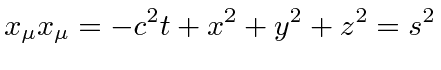An important dot product is that of the difference between two spacetime points. The dot product above gives the distance''in Minkowski space from the origin.

The difference between spacetime points for a single particle is an important case. We use the dot product of this difference with itself.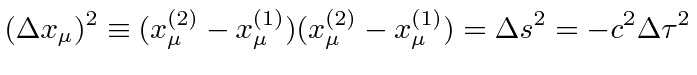The time difference in the particles rest frame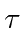is called the proper time and is demonstrated to be a scalar quantity in the above equation.

We define the velocity 4-vector with the equation.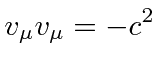We define the momentum 4-vector with.We have shown that in the non-relativistic limit, the 4-momentum is consistent with.We accept this as being the components of the momentum 4-vector giving us the dot product of the momentum 4-vector with itself.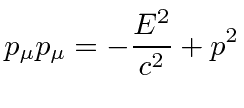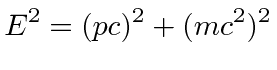The dot product of the momentum 4-vector and the position 4-vectoris related to the phase of waves. For example in quantum mechanics, a free particle with a definite momentum is represented by the plane wave.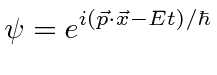We define the Force 4-vector.Jim Branson 2012-10-21